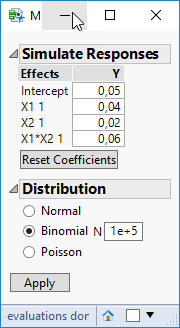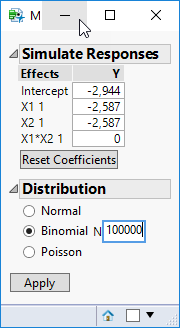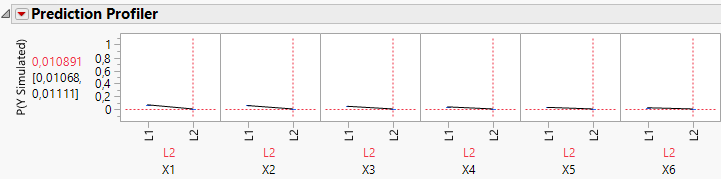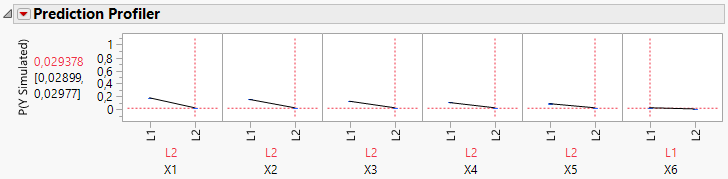It’s World Statistics Day! To honor the theme of the day, the JMP User Community is having conversations about the importance of trust in statistics and data. And we want to hear from you! Tell us the steps you take to ensure that your data is trustworthy.
Choose Language Hide Translation Bar
Highlighted

## JMP Simulate Responses feature for binomial data

Hello!

I want to simulate responses using built in JMP Simulate Responses feature.

I use binomial distribution and my model for simplisity lets say is

p = 0,05 + 0.04*X1 + 0,02*X2.

(Actually I want to use simulation for fractional factorial designs.)

So

 X1 X2 Y -1 -1 0.05 -1 1 0.02 1 -1 0.04 1 1 0.06

In order to simulate this model what values do I need to enter in a Simulate Responses dialog box? Do I need to enter betas? But how do I derive them from coefficients?

Do I need to modify the formula?```Random Binomial(
100000,
1 / (1 + Exp(
-1 * (0.05 + Match( :X1, "-1", 0.04, "1", -0.04, . ) + Match( :X2, "-1", 0.02, "1", -0.02, . )
+Match( :X1, "-1", Match( :X2, "-1", 0.06, "1", -0.06, . ), "1", Match( :X2, "-1", -0.06, "1", 0.06, . ), . ))
))
)```

Learning DOE
7 REPLIES 7
Highlighted

## Re: JMP Simulate Responses feature for binomial data

This discussion might help.

Highlighted

## Re: JMP Simulate Responses feature for binomial data

Hi @gustavjung,

Just to be clear, the linear portion of the a logistic regression model does not directly output probabilities, but the log-odds.  You need to apply the sigmoid (logistic) function (1/(1+exp(-x))) to convert log-odds to probabilities.  That's why the function you posted is 1/(1+e^(-(beta0 + beta1*x1 + beta2*x2 + beta3*x1*x2))).

Coefficients are the betas.  You should input 0 for x1x2 since your desired model has no x1x2 interaction.

-- Cameron Willden
Highlighted

## Re: JMP Simulate Responses feature for binomial data

Thank you very much for your help!

So now I understand that beta is calculated as folows:

p = 0,05 + 0.04*X1 + 0,02*X2.

Intercept (when all levels at -1 is 5% success rate)

is

ln(0,05/1-0,05) = -2,944

X1 (at -1 change from 0.05 to 0.09 at +1)  which is 80% uplift

This is the same as saying that it is an uplift of 40% versus the average response for both levels of the factor.

Which means p = 0.05-40% versus p = 0.05+40%.

p = 0.03 versus 0.07

Which means log odds for X1 = ln( 0.07 / 1-0.07) = -2,587

For X2 (at -1 change from 0.05 to 0.07 at +1)  which is 40% uplift

p = (0.05-20%)=0.04 versus (0.05+20%)=0.06

Which means log odds for X1 = ln( 0.07 / 1-0.07) = -2,752

So I enter this values to Response SimulatorWhen I analyze the simulated data in Generalized Linear Model

I get different prediction responses from that I was expected

Could you please point me on my mistakes?

I have attached the project file if needed.

Learning DOE
Highlighted

## Re: JMP Simulate Responses feature for binomial data

Hi,

I think the problem might be that the intercept is not where all Xs are at -1. Rather, I think the intercept is where all Xs are at 0.

I haven't checked this but you might want to take a look and see if that makes sense.

Phil

Highlighted

## Re: JMP Simulate Responses feature for binomial data

Hi @gustavjung,

JMP parameterizes linear models a bit differently than what most people are used to.  The intercept is the average response (i.e. log-odds) across all factors, so as @phil_kay pointed out, it's actually when the values of X1 and X2 are 0.

If you want P( y = 1 | x1 = -1 & x2 = -1) = 0.05, then you need to basically need to input any combination of beta0, beta1, and beta2 such that beta0 + beta1*(-1) + beta2*(-1) = -2.944.  Obviously, there's infinite solutions, so just plug in whatever you want for 2 of the betas and solve for the last.

-- Cameron Willden
Highlighted

## Re: JMP Simulate Responses feature for binomial data

Hi @cwillden,

Thank you very much for the clarification!

But how do I calculate b1 and b2 if I have calculated b0?

Also I have tried to reproduce this simulation from help site:

https://www.jmp.com/support/help/14/conduct-prospective-power-analysis-for-a-nonline.shtml

However, I couldn't get those numbers in prediction profiler:

 Factor Percent Passing at Xi = 1 Percent Passing at Xi = -1 Difference X1 73.11% 26.89% 46.2% X2 71.09% 28.91% 42.2% X3 69.00% 31.00% 38.0% X4 66.82% 33.18% 33.6% X5 64.56% 35.43% 29.1% X6 62.25% 37.75% 24.5%

In Prediction Profiler

X6 at -1 is 0.010891X6 at +1 is 0.029How does it correspond to initial values?

 X6 62.25% 37.75% 24.5%
Learning DOE
Highlighted

## Re: JMP Simulate Responses feature for binomial data

While you might set the random seed for generating the design, that doesn't apply to simulating responses.  Therefore, you are going to get different results in your fitted model to the simulated data.  If you look at the parameter estimates, they should very roughly correspond to the coefficients you inputted into the simulation.

-- Cameron Willden
Article Labels

There are no labels assigned to this post.